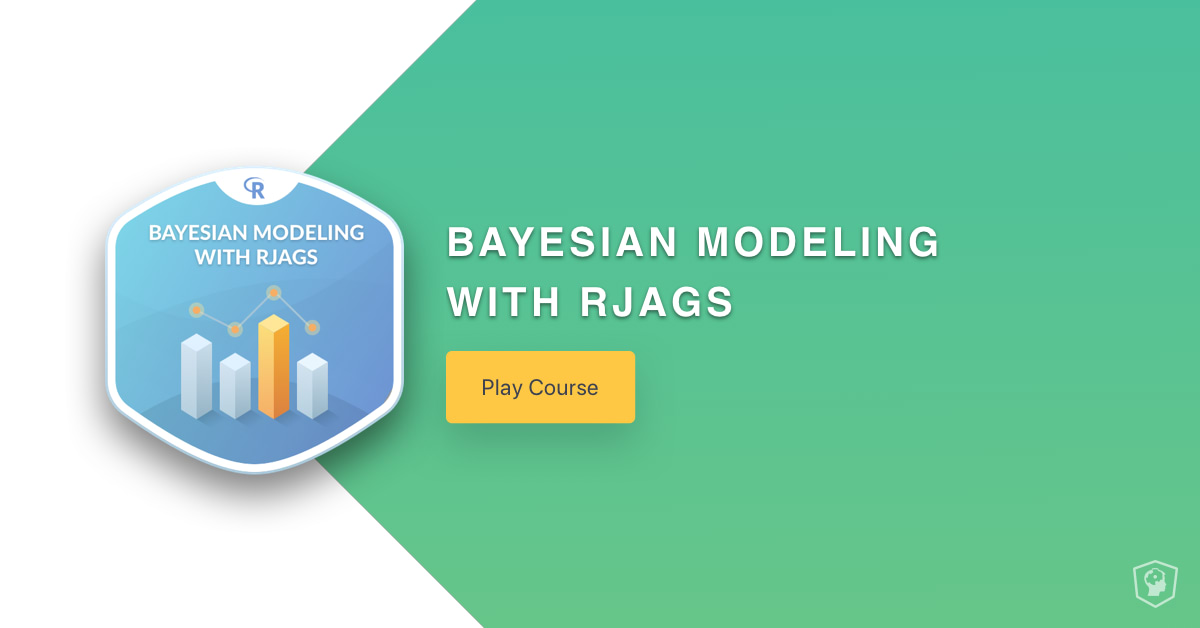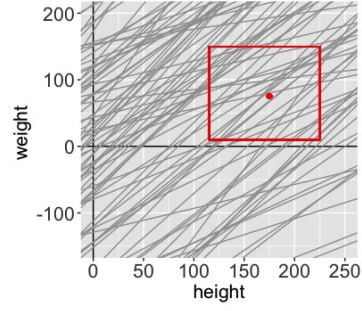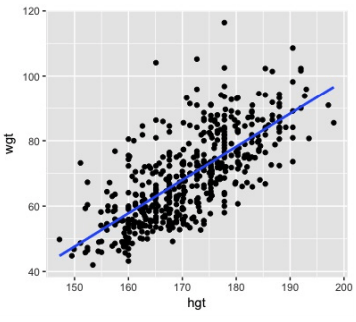Official Blog
r programming
+3

# New Course: Bayesian Modeling with RJAGS

Learn about our new R Course.### Course Description

The Bayesian approach to statistics and machine learning is logical, flexible, and intuitive. In this course, you will engineer and analyze a family of foundational, generalizable Bayesian models. These range in scope from fundamental one-parameter models to intermediate multivariate & generalized linear regression models. The popularity of such Bayesian models has grown along with the availability of computing resources required for their implementation. You will utilize one of these resources - the rjags package in R. Combining the power of R with the JAGS (Just Another Gibbs Sampler) engine, rjags provides a framework for Bayesian modeling, inference, and prediction.

### Chapter 1: Introduction to Bayesian Modeling (Free)

Bayesian models combine prior insights with insights from observed data to form updated, posterior insights about a parameter. In this chapter, you will review these Bayesian concepts in the context of the foundational Beta-Binomial model for a proportion parameter. You will also learn how to use the rjags package to define, compile, and simulate this model in R.### Chapter 2: Bayesian Models & Markov Chains

The two-parameter Normal-Normal Bayesian model provides a simple foundation for Normal regression models. In this chapter, you will engineer the Normal-Normal and define, compile, and simulate this model using rjags. You will also explore the magic of the Markov chain mechanics behind rjags simulation.### Chapter 3: Bayesian Inference & Prediction

In this chapter, you will extend the Normal-Normal model to a simple Bayesian regression model. Within this context, you will explore how to use rjags simulation output to conduct posterior inference. Specifically, you will construct posterior estimates of regression parameters using posterior means & credible intervals, you will test hypotheses using posterior probabilities, and you will construct posterior predictive distributions for new observations.### Chapter 4: Multivariate & Generalized Linear Models

In this final chapter, you will generalize the simple Normal regression model for application in broader contexts. You will incorporate categorical predictors, engineer a multivariate regression model with two predictors, and finally extend this methodology to Poisson multivariate regression models for count variables.